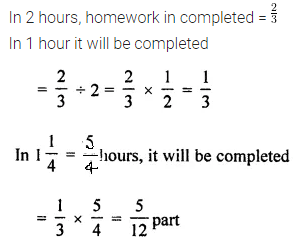# ML Aggarwal Class 7 Solutions for ICSE Maths Chapter 2 Fractions and Decimals Ex 2.4

## ML Aggarwal Class 7 Solutions for ICSE Maths Chapter 2 Fractions and Decimals Ex 2.4

Question 1.
Find the reciprocal of each of the following:
(i) $$\frac { 3 }{ 7 }$$
(iii) $$\frac { 13 }{ 9 }$$
(iii) 8
Solution: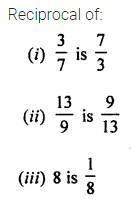Question 2.
Evaluate the following: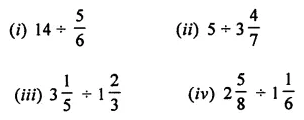Solution: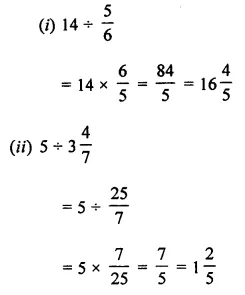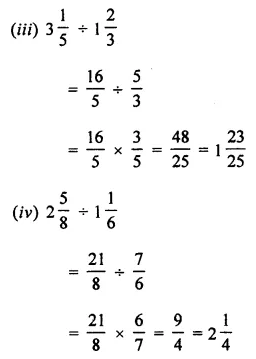Question 3.
How many pieces each 5$$\frac { 1 }{ 6 }$$ metres long can be cut from a cloth 77$$\frac { 1 }{ 2 }$$ metres long?
Solution:Question 4.
By what number should 4$$\frac { 7 }{ 8 }$$ be multiplied to get 87$$\frac { 3 }{ 4 }$$ ?
Solution: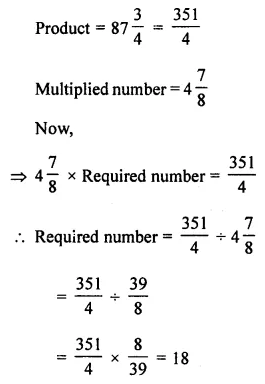Question 5.
In a hostel’s mess, each student gets $$\frac { 1 }{ 3 }$$ litre of milk every day. If the total consumption of the milk is 57$$\frac { 2 }{ 3 }$$ litres per day, how many students are there in the hostel?
Solution: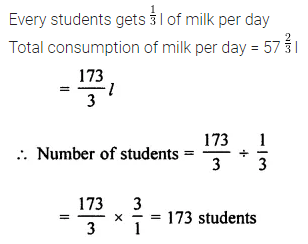Question 6.
The cost of 5$$\frac { 1 }{ 4 }$$ kg apples is ₹ 336. What is the rate of apples per kg?
Solution:Question 7.
The length of a rectangular plot of area 68$$\frac { 3 }{ 4 }$$ sq. m is 12$$\frac { 1 }{ 2 }$$ m, find its width.
Solution: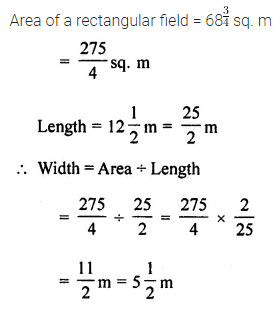Question 8.
If the cost of 5$$\frac { 1 }{ 2 }$$ kg of sugar is ₹ 206$$\frac { 1 }{ 4 }$$,then find the cost of 8$$\frac { 1 }{ 4 }$$ kg of sugar.
Solution:Question 9.
Renu completed $$\frac { 2 }{ 3 }$$ part of her homework in 2 hours. How much part of her homework had she completed in 1$$\frac { 1 }{ 4 }$$ hours?
Solution: